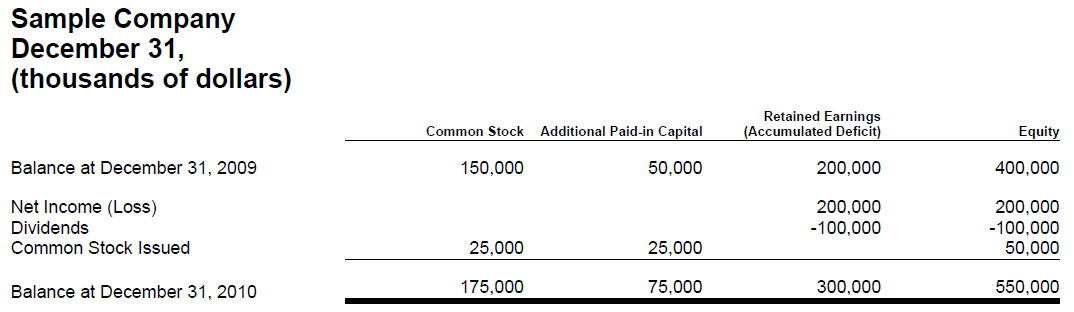# Information Model Metapatterns Summary

Metapatterns documentation (PDF)

Visual Index of Metapatterns

# Metapattern name Description Visual image
1. Hierarchy A hierarchy information model denotes a hierarchy of concepts with no numeric relations. If no numeric relations exist, then the information model of the component is a hierarchy. Basically, anything can be modelled as a hierarchy. It is the addition of additional relations, typically computations, which turns a hierarchy into some other metapattern.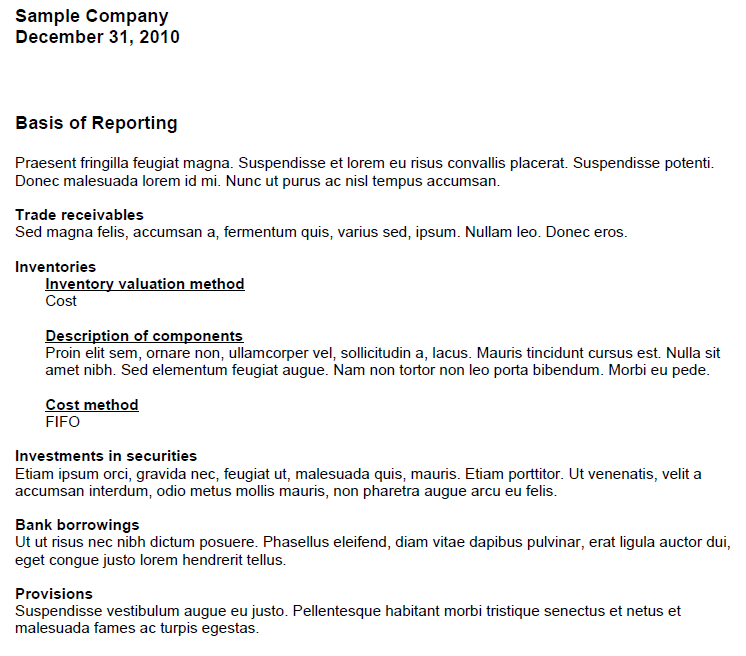2. Roll Up A roll up information model computes a total from a set of other concepts. This information model is commonly referred to a “roll up”, or by the equation A + B = C. All concepts involved in this information model have the same set of characteristics and all must be numeric.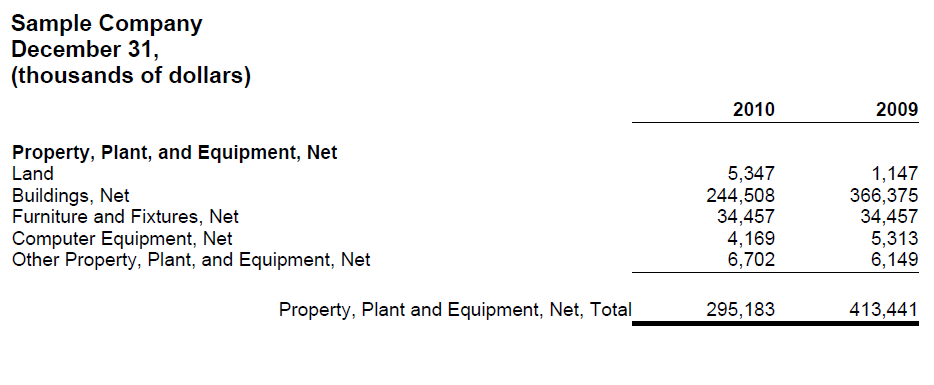3. Roll Forward A roll forward information model reconciles the balance of a concept between two points in time. This information model is commonly referred to a “roll forward” or “movement analysis” or by the equation: beginning balance + changes = ending balance. In this equation period [Axis] is as of two different points in time and the changes occur during the period between those two points in time.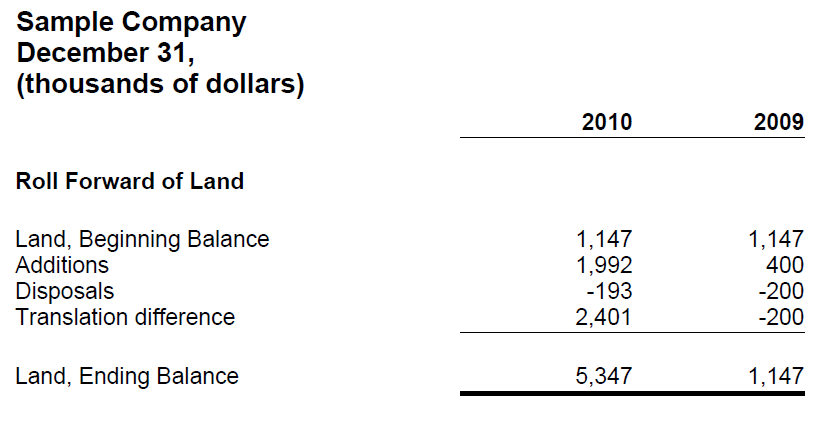4. Compound Fact A compound fact information model is characterized by the fact that for some set of concepts expressed within some information model; that information model can be expressed over some characteristic expressed as an [Axis]. Basically, it is the [Axis] which provides additional information which makes each information model unique. For example, the salary information for the directors of an entity is a compound fact. The salary information is made up of salary, bonuses, director fees and such information must be associated with a specific director to be meaningful and to distinguish, say, one salary from another salary.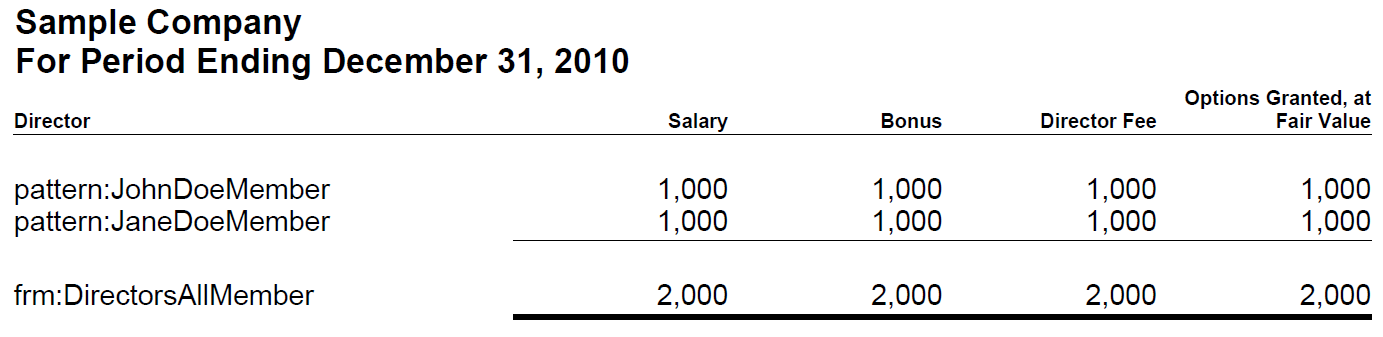5. Adjustment An adjustment information model reconciles an originally stated balance to a restated balance, the adjustment being the total change, between two different report dates. An adjustment is similar to a roll forward in that it is a reconciliation, however rather than the period [Axis] changing; it is the Report Date [Axis] which changes: originally reported balance + adjustment = restated balance.6. Variance A variance information model reconciles some reporting scenario with another reporting scenario, the variance between reporting scenarios being the variance or changes. For example, a sales analysis which reconciles the concept sales for the reporting scenarios of actual and budgeted is a variance. The equation in this case is: actual – budget = variance. But a variance could take other forms such as a variance from forecast, variance from plan, etc.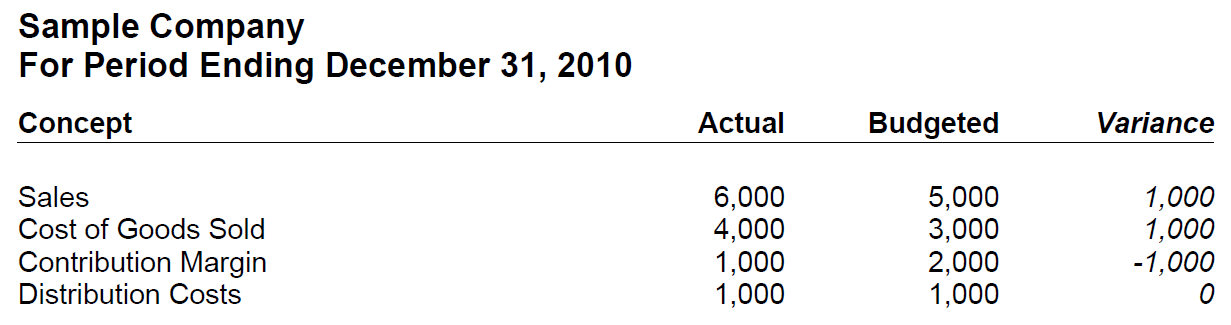7. Complex Computation A complex computation information model can be thought of as a hierarchy plus a set of commutations between different concepts within that hierarchy which are more challenging to model than a roll up or roll forward. The type of computations can vary significantly, thus the challenging in modelling. For example, the computation of earnings per share is a complex computation.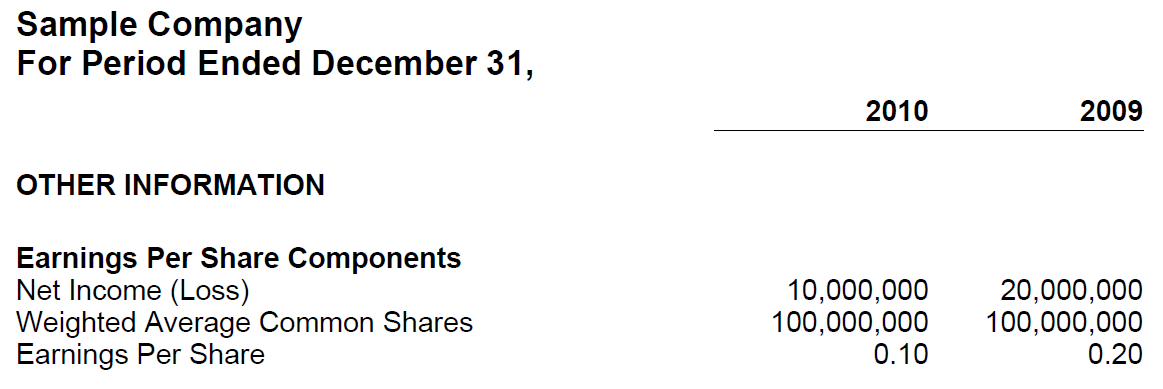8. Text Block A text block information model is an information model which contains, by definition, only one concept and that concept expresses what amounts to a narrative or prose as escaped HTML within that one concept. For example, the narrative associated with a set of accounting policies expressed as a list or a table presentation format is a text block. As there is only one concept, there can be no relations within the information model.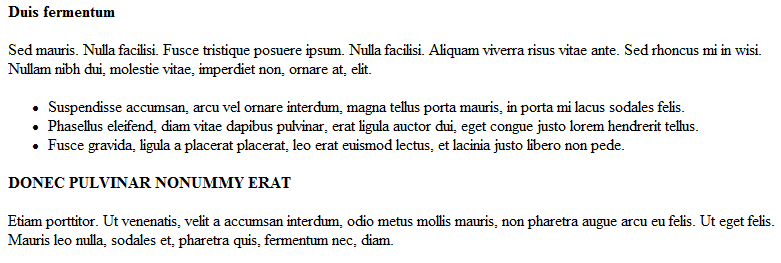9. Grid A grid information model is a pseudo metapattern which uses the presentation characteristics of the columns and rows of a table to model information. Because the grid models presentation information and not business semantics, it cannot be considered a metapattern. However, the grid is included in this list because the US GAAP Taxonomy uses a grid information model to model the statement of changes in equity.